## Friday, December 8, 2017

Finding the number of Triangles and Squares in the given pictures is quite Good Visualization Fun Puzzles. It helps to enhance you attention as one has to concentrate a lot while differentiating one unit of triangle or square from the another one. Moreover one has to also take care of Triangles or Squares which get created during the overlapping of pictures.  Here I am posting some of the picture in which you have to find number of Triangles and Squares. Do post you comments giving your count of found out triangles and squares in these pictures. These riddles are part of Fun Brain Teasers and Riddles.

1. Can you find how many squares are there in this figure. In fact this brain teaser is for kids as it is quite easy. Moreover because of colors of different squares it becomes even easier to find number of squares.1. How Many Squares can you count in this Picture?

This is quite easy one. Now try another find number of Squares in Figure which is little tough one.
2. Here is the another picture in which one has to find number of squares. Again this is an easy one. However try to count it correctly in the first try itself. I could not answer it correctly in first try. Lets see if you can do it?2. How Many Squares in this Puzzle Picture?

3. The Next one is harder one as compared to previous two. As there is overlapping of squares. In this picture again one has to find number of Squares. Do remember that a Square is always a Rectangle. However Rectangle is not always a square.3. How Many Square can you count?

4. Finding Squares in a figure is easier than finding triangles in a figure. In the next few puzzles one has to find number of Triangles in the given picture. Starting with the first one, lets try to find number of triangles in this photograph. Do take care that there will overlapping of Triangles at many places. So one has to be very careful while counting number of triangles in the given following picture.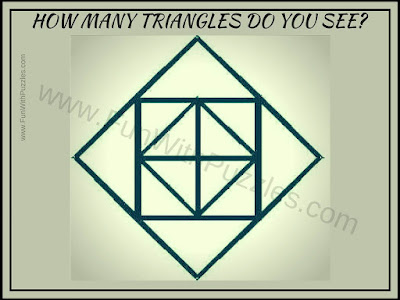4. How Many Triangles can you count in this Picture?

5. It is time now to move slowly to  tough and complex pictures. Here is one picture of triangle which has many triangles inside it. Can you count all the triangles which you see in this picture puzzle image?5. How many triangles you can find in this Picture?

6. Lets move the complex picture of finding number of triangles. This picture contains lots of overlapped triangles. Lets see how many triangles you can count in this complex picture?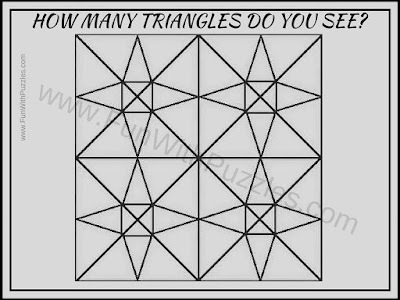6. How Many Triangles Do you see in this picture?

Do post you comments while answering these puzzles. Lets see how correctly you can find these triangles and squares?

Also do checkout below mentioned interesting and challenging Picture Puzzles
List of Interesting and Challenging Picture Puzzles

1. Easy Picture Puzzles to count Number of Triangles: Another set of Picture Puzzles which are easy puzzles in which your challenge is to count number of triangles.

2. Brain Teasers Pictures Puzzles with answers: It contains different types of Puzzle Pictures Brain Teasers which will test your observation skills.

3. Intelligent Reading Riddles to Twist your brain: After all these counting picture puzzles, its time now to take the reading challenge. Can you read it?

4. Can you find the mistake in these pictures: Test you visual and observational mind power by solving these picture puzzles in which your challenge is to find the mistakes in given puzzle images.

<<<<<<<<<<<<>>>>>>>>>>>>

Here is answer to 8th Brain Teaser Question of post titled "Out of Box Thinking Fun Brain Teasers with answers to Challenge your Mind".
I have hidden this answer to avoid any spoiler. Do select the text below to read the answer.
>>>>>>>>>>>>>>>>>>

Whatsapp

I told in the hint that this is many of us use it everyday and may be in few years something new will come and we all will forget this application. How many of us remember Orkut?

<<<<<<<<<<<<<<<<<<

1.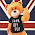8 or 9?

2.how many triangles in puzzle 6? is it 120?

1.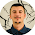3.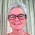The answer to the second one is 0

4.the answer to #2 is none those "squares" are rectangles

5.